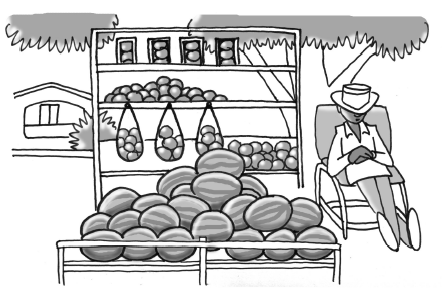Home > CCAA8 > Chapter cca10 > Lesson cca10.3.2 > Problem10-126

10-126.Solve the following problem and write your solution as a sentence. Homework Help ✎

Mr. Ripley’s fruit stand sells watermelons for $\5$ and apples for $\2$. Last weekend, he sold $40$ pieces of fruit (all apples and watermelons) for $\107$. How many watermelons did he sell?

Write two equations. One equation for the number of fruit and one equation for the cost of the fruit.
Use this system of equations to find the number of watermelons Mr. Ripley sold.

He sold $9$ watermelons.